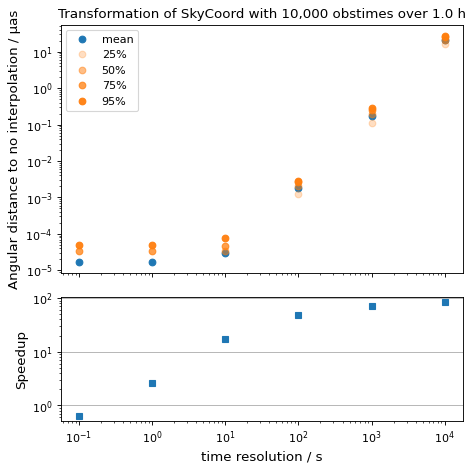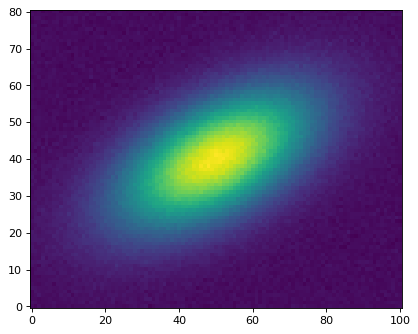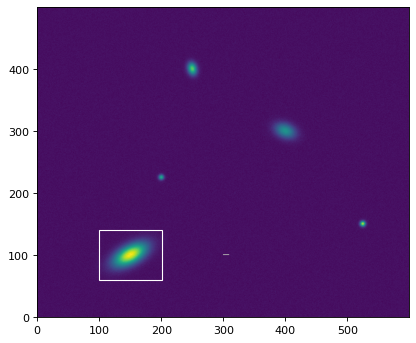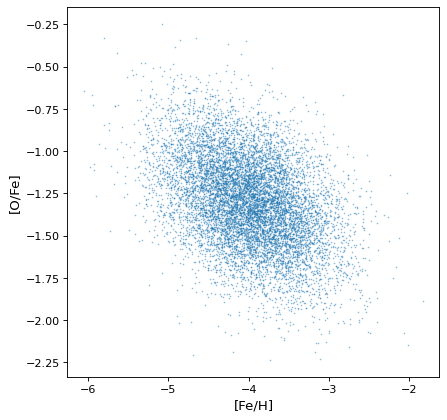# Models and Fitting (astropy.modeling)¶

## Introduction¶

astropy.modeling provides a framework for representing models and performing model evaluation and fitting. It currently supports 1-D and 2-D models and fitting with parameter constraints.

It is designed to be easily extensible and flexible. Models do not reference fitting algorithms explicitly and new fitting algorithms may be added without changing the existing models (though not all models can be used with all fitting algorithms due to constraints such as model linearity).

The goal is to eventually provide a rich toolset of models and fitters such that most users will not need to define new model classes, nor special purpose fitting routines (while making it reasonably easy to do when necessary).

Note

astropy.modeling is currently a work-in-progress, and thus it is likely there will still be API changes in later versions of Astropy. Backwards compatibility support between versions will still be maintained as much as possible, but new features and enhancements are coming in future versions. If you have specific ideas for how it might be improved, feel free to let us know on the astropy-dev mailing list or at http://feedback.astropy.org

## Getting started¶

The examples here use the predefined models and assume the following modules have been imported:

>>> import numpy as np
>>> from astropy.modeling import models, fitting


### Using Models¶

The astropy.modeling package defines a number of models that are collected under a single namespace as astropy.modeling.models. Models behave like parametrized functions:

>>> from astropy.modeling import models
>>> g = models.Gaussian1D(amplitude=1.2, mean=0.9, stddev=0.5)
>>> print(g)
Model: Gaussian1D
Inputs: ('x',)
Outputs: ('y',)
Model set size: 1
Parameters:
amplitude mean stddev
--------- ---- ------
1.2  0.9    0.5


Model parameters can be accessed as attributes:

>>> g.amplitude
Parameter('amplitude', value=1.2)
>>> g.mean
Parameter('mean', value=0.9)
>>> g.stddev
Parameter('stddev', value=0.5, bounds=(1.1754943508222875e-38, None))


and can also be updated via those attributes:

>>> g.amplitude = 0.8
>>> g.amplitude
Parameter('amplitude', value=0.8)


Models can be evaluated by calling them as functions:

>>> g(0.1)
0.22242984036255528
>>> g(np.linspace(0.5, 1.5, 7))
array([0.58091923, 0.71746405, 0.7929204 , 0.78415894, 0.69394278,
0.54952605, 0.3894018 ])


As the above example demonstrates, in general most models evaluate array-like inputs according to the standard Numpy broadcasting rules for arrays.

Models can therefore already be useful to evaluate common functions, independently of the fitting features of the package.

### Simple 1-D model fitting¶

In this section, we look at a simple example of fitting a Gaussian to a simulated dataset. We use the Gaussian1D and Trapezoid1D models and the LevMarLSQFitter fitter to fit the data:

import numpy as np
import matplotlib.pyplot as plt
from astropy.modeling import models, fitting

# Generate fake data
np.random.seed(0)
x = np.linspace(-5., 5., 200)
y = 3 * np.exp(-0.5 * (x - 1.3)**2 / 0.8**2)
y += np.random.normal(0., 0.2, x.shape)

# Fit the data using a box model.
# Bounds are not really needed but included here to demonstrate usage.
t_init = models.Trapezoid1D(amplitude=1., x_0=0., width=1., slope=0.5,
bounds={"x_0": (-5., 5.)})
fit_t = fitting.LevMarLSQFitter()
t = fit_t(t_init, x, y)

# Fit the data using a Gaussian
g_init = models.Gaussian1D(amplitude=1., mean=0, stddev=1.)
fit_g = fitting.LevMarLSQFitter()
g = fit_g(g_init, x, y)

# Plot the data with the best-fit model
plt.figure(figsize=(8,5))
plt.plot(x, y, 'ko')
plt.plot(x, t(x), label='Trapezoid')
plt.plot(x, g(x), label='Gaussian')
plt.xlabel('Position')
plt.ylabel('Flux')
plt.legend(loc=2)


()As shown above, once instantiated, the fitter class can be used as a function that takes the initial model (t_init or g_init) and the data values (x and y), and returns a fitted model (t or g).

### Simple 2-D model fitting¶

Similarly to the 1-D example, we can create a simulated 2-D data dataset, and fit a polynomial model to it. This could be used for example to fit the background in an image.

import warnings
import numpy as np
import matplotlib.pyplot as plt
from astropy.modeling import models, fitting

# Generate fake data
np.random.seed(0)
y, x = np.mgrid[:128, :128]
z = 2. * x ** 2 - 0.5 * x ** 2 + 1.5 * x * y - 1.
z += np.random.normal(0., 0.1, z.shape) * 50000.

# Fit the data using astropy.modeling
p_init = models.Polynomial2D(degree=2)
fit_p = fitting.LevMarLSQFitter()

with warnings.catch_warnings():
# Ignore model linearity warning from the fitter
warnings.simplefilter('ignore')
p = fit_p(p_init, x, y, z)

# Plot the data with the best-fit model
plt.figure(figsize=(8, 2.5))
plt.subplot(1, 3, 1)
plt.imshow(z, origin='lower', interpolation='nearest', vmin=-1e4, vmax=5e4)
plt.title("Data")
plt.subplot(1, 3, 2)
plt.imshow(p(x, y), origin='lower', interpolation='nearest', vmin=-1e4,
vmax=5e4)
plt.title("Model")
plt.subplot(1, 3, 3)
plt.imshow(z - p(x, y), origin='lower', interpolation='nearest', vmin=-1e4,
vmax=5e4)
plt.title("Residual")


()A list of models is provided in the Reference/API section. The fitting framework includes many useful features that are not demonstrated here, such as weighting of datapoints, fixing or linking parameters, and placing lower or upper limits on parameters. For more information on these, take a look at the Fitting Models to Data documentation.

### Model sets¶

In some cases it is necessary to describe many models of the same type but with different sets of parameter values. This could be done simply by instantiating as many instances of a Model as are needed. But that can be inefficient for a large number of models. To that end, all model classes in astropy.modeling can also be used to represent a model set which is a collection of models of the same type, but with different values for their parameters.

To instantiate a model set, use argument n_models=N where N is the number of models in the set when constructing the model. The value of each parameter must be a list or array of length N, such that each item in the array corresponds to one model in the set:

>>> g = models.Gaussian1D(amplitude=[1, 2], mean=[0, 0],
...                       stddev=[0.1, 0.2], n_models=2)
>>> print(g)
Model: Gaussian1D
Inputs: ('x',)
Outputs: ('y',)
Model set size: 2
Parameters:
amplitude mean stddev
--------- ---- ------
1.0  0.0    0.1
2.0  0.0    0.2


This is equivalent to two Gaussians with the parameters amplitude=1, mean=0, stddev=0.1 and amplitude=2, mean=0, stddev=0.2 respectively. When printing the model the parameter values are displayed as a table, with each row corresponding to a single model in the set.

The number of models in a model set can be determined using the len builtin:

>>> len(g)
2


Single models have a length of 1, and are not considered a model set as such.

When evaluating a model set, by default the input must be the same length as the number of models, with one input per model:

>>> g([0, 0.1])
array([1.        , 1.76499381])


The result is an array with one result per model in the set. It is also possible to broadcast a single value to all models in the set:

>>> g(0)
array([1., 2.])


Model sets are used primarily for fitting, allowing a large number of models of the same type to be fitted simultaneously (and independently from each other) to some large set of inputs. For example, fitting a polynomial to the time response of each pixel in a data cube. This can greatly speed up the fitting process, especially for linear models.

### Compound models¶

New in version 1.0: This feature is experimental and expected to see significant further development, but the basic usage is stable and expected to see wide use.

While the Astropy modeling package makes it very easy to define new models either from existing functions, or by writing a Model subclass, an additional way to create new models is by combining them using arithmetic expressions. This works with models built into Astropy, and most user-defined models as well. For example, it is possible to create a superposition of two Gaussians like so:

>>> from astropy.modeling import models
>>> g1 = models.Gaussian1D(1, 0, 0.2)
>>> g2 = models.Gaussian1D(2.5, 0.5, 0.1)
>>> g1_plus_2 = g1 + g2


The resulting object g1_plus_2 is itself a new model. Evaluating, say, g1_plus_2(0.25) is the same as evaluating g1(0.25) + g2(0.25):

>>> g1_plus_2(0.25)
0.5676756958301329
>>> g1_plus_2(0.25) == g1(0.25) + g2(0.25)
True


This model can be further combined with other models in new expressions.

These new compound models can also be fitted to data, like most other models (though this currently requires one of the non-linear fitters):

import numpy as np
import matplotlib.pyplot as plt
from astropy.modeling import models, fitting

# Generate fake data
np.random.seed(42)
g1 = models.Gaussian1D(1, 0, 0.2)
g2 = models.Gaussian1D(2.5, 0.5, 0.1)
x = np.linspace(-1, 1, 200)
y = g1(x) + g2(x) + np.random.normal(0., 0.2, x.shape)

# Now to fit the data create a new superposition with initial
# guesses for the parameters:
gg_init = models.Gaussian1D(1, 0, 0.1) + models.Gaussian1D(2, 0.5, 0.1)
fitter = fitting.SLSQPLSQFitter()
gg_fit = fitter(gg_init, x, y)

# Plot the data with the best-fit model
plt.figure(figsize=(8,5))
plt.plot(x, y, 'ko')
plt.plot(x, gg_fit(x))
plt.xlabel('Position')
plt.ylabel('Flux')


()This works for 1-D models, 2-D models, and combinations thereof, though there are some complexities involved in correctly matching up the inputs and outputs of all models used to build a compound model. You can learn more details in the Compound Models documentation.

Astropy models also support convolution through the function convolve_models, which returns a compound model.

For instance, the convolution of two Gaussian functions is also a Gaussian function in which the resulting mean (variance) is the sum of the means (variances) of each Gaussian.

import numpy as np
import matplotlib.pyplot as plt
from astropy.modeling import models
from astropy.convolution import convolve_models

g1 = models.Gaussian1D(1, -1, 1)
g2 = models.Gaussian1D(1, 1, 1)
g3 = convolve_models(g1, g2)

x = np.linspace(-3, 3, 50)
plt.plot(x, g1(x), 'k-')
plt.plot(x, g2(x), 'k-')
plt.plot(x, g3(x), 'k-')


()New in version 2.0.4.

When astropy.modeling.fitting.LinearLSQFitter is provided with the dependent co-ordinate values as a numpy.ma.MaskedArray, it ignores any masked values when performing the fit:

>>> p_init = models.Polynomial1D(degree=1)
>>> x = np.arange(10)
>>> y = 100.       # simulate spurious value
>>> fitter = fitting.LinearLSQFitter()
>>> p = fitter(p_init, x, y)
>>> print('Fit intercept={:.3f}, slope={:.3f}'.format(p.c0.value, p.c1.value))
Fit intercept=1.000, slope=2.000


At present, the non-linear fitters do not distinguish between good and bad values in this way.

Note that model set fitting is currently about an order of magnitude slower in the presence of masked values, because the matrix equation has to be solved for each model separately, on their respective co-ordinate grids. This is still an order of magnitude faster than fitting separate model instances, however. Supplying a numpy.ma.MaskedArray without any bad (True) mask values produces the normal, faster behavior.

## Performance Tips¶

Initializing a compound model with many constituent models can be time consuming. If your code uses the same compound model repeatedly consider initializing it once and reusing the model.

Consider the performance tips that apply to quantities when initializing and evaluating models with quantities.

## Reference/API¶

### astropy.modeling Package¶

This subpackage provides a framework for representing models and performing model evaluation and fitting. It supports 1D and 2D models and fitting with parameter constraints. It has some predefined models and fitting routines.

#### Functions¶

 custom_model(\*args[, fit_deriv]) Create a model from a user defined function. is_separable(transform) A separability test for the outputs of a transform. separability_matrix(transform) Compute the correlation between outputs and inputs.

#### Classes¶

 Fittable1DModel(*args[, meta, name]) Base class for one-dimensional fittable models. Fittable2DModel(*args[, meta, name]) Base class for two-dimensional fittable models. FittableModel(*args[, meta, name]) Base class for models that can be fitted using the built-in fitting algorithms. InputParameterError Used for incorrect input parameter values and definitions. Model(*args[, meta, name]) Base class for all models. ModelDefinitionError Used for incorrect models definitions Parameter([name, description, default, …]) Wraps individual parameters. ParameterError Generic exception class for all exceptions pertaining to Parameters.

### astropy.modeling.functional_models Module¶

Mathematical models.

#### Classes¶

 AiryDisk2D([amplitude, x_0, y_0, radius]) Two dimensional Airy disk model. Moffat1D([amplitude, x_0, gamma, alpha]) One dimensional Moffat model. Moffat2D([amplitude, x_0, y_0, gamma, alpha]) Two dimensional Moffat model. Box1D([amplitude, x_0, width]) One dimensional Box model. Box2D([amplitude, x_0, y_0, x_width, y_width]) Two dimensional Box model. Const1D([amplitude]) One dimensional Constant model. Const2D([amplitude]) Two dimensional Constant model. Ellipse2D([amplitude, x_0, y_0, a, b, theta]) A 2D Ellipse model. Disk2D([amplitude, x_0, y_0, R_0]) Two dimensional radial symmetric Disk model. Gaussian1D([amplitude, mean, stddev]) One dimensional Gaussian model. Gaussian2D([amplitude, x_mean, y_mean, …]) Two dimensional Gaussian model. Linear1D([slope, intercept]) One dimensional Line model. Lorentz1D([amplitude, x_0, fwhm]) One dimensional Lorentzian model. MexicanHat1D([amplitude, x_0, sigma]) One dimensional Mexican Hat model. MexicanHat2D([amplitude, x_0, y_0, sigma]) Two dimensional symmetric Mexican Hat model. One dimensional redshift scale factor model. Multiply([factor]) Multiply a model by a quantity or number. Planar2D([slope_x, slope_y, intercept]) Two dimensional Plane model. Scale([factor]) Multiply a model by a dimensionless factor. Sersic1D([amplitude, r_eff, n]) One dimensional Sersic surface brightness profile. Sersic2D([amplitude, r_eff, n, x_0, y_0, …]) Two dimensional Sersic surface brightness profile. Shift([offset]) Shift a coordinate. Sine1D([amplitude, frequency, phase]) One dimensional Sine model. Trapezoid1D([amplitude, x_0, width, slope]) One dimensional Trapezoid model. TrapezoidDisk2D([amplitude, x_0, y_0, R_0, …]) Two dimensional circular Trapezoid model. Ring2D([amplitude, x_0, y_0, r_in, width, r_out]) Two dimensional radial symmetric Ring model. Voigt1D([x_0, amplitude_L, fwhm_L, fwhm_G]) One dimensional model for the Voigt profile.

### astropy.modeling.powerlaws Module¶

Power law model variants

#### Classes¶

 PowerLaw1D([amplitude, x_0, alpha]) One dimensional power law model. BrokenPowerLaw1D([amplitude, x_break, …]) One dimensional power law model with a break. SmoothlyBrokenPowerLaw1D([amplitude, …]) One dimensional smoothly broken power law model. ExponentialCutoffPowerLaw1D([amplitude, …]) One dimensional power law model with an exponential cutoff. LogParabola1D([amplitude, x_0, alpha, beta]) One dimensional log parabola model (sometimes called curved power law).

### astropy.modeling.blackbody Module¶

Model and functions related to blackbody radiation.

Blackbody flux is calculated with Planck law (Rybicki & Lightman 1979):

\begin{align}\begin{aligned}B_{\lambda}(T) = \frac{2 h c^{2} / \lambda^{5}}{exp(h c / \lambda k T) - 1}\\B_{\nu}(T) = \frac{2 h \nu^{3} / c^{2}}{exp(h \nu / k T) - 1}\end{aligned}\end{align}

where the unit of $$B_{\lambda}(T)$$ is $$erg \; s^{-1} cm^{-2} \mathring{A}^{-1} sr^{-1}$$, and $$B_{\nu}(T)$$ is $$erg \; s^{-1} cm^{-2} Hz^{-1} sr^{-1}$$. blackbody_lambda() and blackbody_nu() calculate the blackbody flux for $$B_{\lambda}(T)$$ and $$B_{\nu}(T)$$, respectively.

For blackbody representation as a model, see BlackBody1D.

##### Examples¶
>>> import numpy as np
>>> from astropy import units as u
>>> from astropy.modeling.blackbody import blackbody_lambda, blackbody_nu


Calculate blackbody flux for 5000 K at 100 and 10000 Angstrom while suppressing any Numpy warnings:

>>> wavelengths = [100, 10000] * u.AA
>>> temperature = 5000 * u.K
>>> with np.errstate(all='ignore'):
...     flux_lam = blackbody_lambda(wavelengths, temperature)
...     flux_nu = blackbody_nu(wavelengths, temperature)
>>> flux_lam
<Quantity [  1.27452545e-108,  7.10190526e+005] erg / (Angstrom cm2 s sr)>
>>> flux_nu
<Quantity [  4.25135927e-123,  2.36894060e-005] erg / (cm2 Hz s sr)>


Alternatively, the same results for flux_nu can be computed using BlackBody1D with blackbody representation as a model. The difference between this and the former approach is in one additional step outlined as follows:

>>> from astropy import constants as const
>>> from astropy.modeling import models
>>> temperature = 5000 * u.K
>>> bolometric_flux = const.sigma_sb * temperature ** 4 / np.pi
>>> bolometric_flux.to(u.erg / (u.cm * u.cm * u.s))
<Quantity 1.12808367e+10 erg / (cm2 s)>
>>> wavelengths = [100, 10000] * u.AA
>>> bb_astro = models.BlackBody1D(temperature, bolometric_flux=bolometric_flux)
>>> bb_astro(wavelengths).to(u.erg / (u.cm * u.cm * u.Hz * u.s)) / u.sr
<Quantity [4.25102471e-123, 2.36893879e-005] erg / (cm2 Hz s sr)>


where bb_astro(wavelengths) computes the equivalent result as flux_nu above.

Plot a blackbody spectrum for 5000 K:

()Note that an array of temperatures can also be given instead of a single temperature. In this case, the Numpy broadcasting rules apply: for instance, if the frequency and temperature have the same shape, the output will have this shape too, while if the frequency is a 2-d array with shape (n, m) and the temperature is an array with shape (m,), the output will have a shape (n, m).

Rybicki, G. B., & Lightman, A. P. 1979, Radiative Processes in Astrophysics (New York, NY: Wiley)

#### Functions¶

 blackbody_nu(in_x, temperature) Calculate blackbody flux per steradian, $$B_{\nu}(T)$$. blackbody_lambda(in_x, temperature) Like blackbody_nu() but for $$B_{\lambda}(T)$$.

#### Classes¶

 BlackBody1D([temperature, bolometric_flux]) One dimensional blackbody model.

### astropy.modeling.polynomial Module¶

This module contains models representing polynomials and polynomial series.

#### Classes¶

 Chebyshev1D(degree[, domain, window, …]) Univariate Chebyshev series. Chebyshev2D(x_degree, y_degree[, x_domain, …]) Bivariate Chebyshev series.. Hermite1D(degree[, domain, window, …]) Univariate Hermite series. Hermite2D(x_degree, y_degree[, x_domain, …]) Bivariate Hermite series. InverseSIP(ap_order, bp_order[, ap_coeff, …]) Inverse Simple Imaging Polynomial Legendre1D(degree[, domain, window, …]) Univariate Legendre series. Legendre2D(x_degree, y_degree[, x_domain, …]) Bivariate Legendre series. Polynomial1D(degree[, domain, window, …]) 1D Polynomial model. Polynomial2D(degree[, x_domain, y_domain, …]) 2D Polynomial model. SIP(crpix, a_order, b_order[, a_coeff, …]) Simple Imaging Polynomial (SIP) model. OrthoPolynomialBase(x_degree, y_degree[, …]) This is a base class for the 2D Chebyshev and Legendre models. PolynomialModel(degree[, n_models, …]) Base class for polynomial models.

### astropy.modeling.projections Module¶

Implements projections–particularly sky projections defined in WCS Paper II .

All angles are set and and displayed in degrees but internally computations are performed in radians. All functions expect inputs and outputs degrees.

#### Classes¶

 Projection(*args[, meta, name]) Base class for all sky projections. Pix2SkyProjection(*args[, meta, name]) Base class for all Pix2Sky projections. Sky2PixProjection(*args[, meta, name]) Base class for all Sky2Pix projections. Zenithal(*args[, meta, name]) Base class for all Zenithal projections. Cylindrical(*args[, meta, name]) Base class for Cylindrical projections. PseudoCylindrical(*args[, meta, name]) Base class for pseudocylindrical projections. Conic(*args[, meta, name]) Base class for conic projections. PseudoConic(*args[, meta, name]) Base class for pseudoconic projections. QuadCube(*args[, meta, name]) Base class for quad cube projections. HEALPix(*args[, meta, name]) Base class for HEALPix projections. AffineTransformation2D([matrix, translation]) Perform an affine transformation in 2 dimensions. Pix2Sky_ZenithalPerspective([mu, gamma]) Zenithal perspective projection - pixel to sky. Sky2Pix_ZenithalPerspective([mu, gamma]) Zenithal perspective projection - sky to pixel. Pix2Sky_SlantZenithalPerspective([mu, phi0, …]) Slant zenithal perspective projection - pixel to sky. Sky2Pix_SlantZenithalPerspective([mu, phi0, …]) Zenithal perspective projection - sky to pixel. Pix2Sky_Gnomonic(*args[, meta, name]) Gnomonic projection - pixel to sky. Sky2Pix_Gnomonic(*args[, meta, name]) Gnomonic Projection - sky to pixel. Pix2Sky_Stereographic(*args[, meta, name]) Stereographic Projection - pixel to sky. Sky2Pix_Stereographic(*args[, meta, name]) Stereographic Projection - sky to pixel. Pix2Sky_SlantOrthographic([xi, eta]) Slant orthographic projection - pixel to sky. Sky2Pix_SlantOrthographic([xi, eta]) Slant orthographic projection - sky to pixel. Pix2Sky_ZenithalEquidistant(*args[, meta, name]) Zenithal equidistant projection - pixel to sky. Sky2Pix_ZenithalEquidistant(*args[, meta, name]) Zenithal equidistant projection - sky to pixel. Pix2Sky_ZenithalEqualArea(*args[, meta, name]) Zenithal equidistant projection - pixel to sky. Sky2Pix_ZenithalEqualArea(*args[, meta, name]) Zenithal equidistant projection - sky to pixel. Pix2Sky_Airy([theta_b]) Airy projection - pixel to sky. Sky2Pix_Airy([theta_b]) Airy - sky to pixel. Pix2Sky_CylindricalPerspective([mu, lam]) Cylindrical perspective - pixel to sky. Sky2Pix_CylindricalPerspective([mu, lam]) Cylindrical Perspective - sky to pixel. Cylindrical equal area projection - pixel to sky. Cylindrical equal area projection - sky to pixel. Pix2Sky_PlateCarree(*args[, meta, name]) Plate carrée projection - pixel to sky. Sky2Pix_PlateCarree(*args[, meta, name]) Plate carrée projection - sky to pixel. Pix2Sky_Mercator(*args[, meta, name]) Mercator - pixel to sky. Sky2Pix_Mercator(*args[, meta, name]) Mercator - sky to pixel. Pix2Sky_SansonFlamsteed(*args[, meta, name]) Sanson-Flamsteed projection - pixel to sky. Sky2Pix_SansonFlamsteed(*args[, meta, name]) Sanson-Flamsteed projection - sky to pixel. Pix2Sky_Parabolic(*args[, meta, name]) Parabolic projection - pixel to sky. Sky2Pix_Parabolic(*args[, meta, name]) Parabolic projection - sky to pixel. Pix2Sky_Molleweide(*args[, meta, name]) Molleweide’s projection - pixel to sky. Sky2Pix_Molleweide(*args[, meta, name]) Molleweide’s projection - sky to pixel. Pix2Sky_HammerAitoff(*args[, meta, name]) Hammer-Aitoff projection - pixel to sky. Sky2Pix_HammerAitoff(*args[, meta, name]) Hammer-Aitoff projection - sky to pixel. Pix2Sky_ConicPerspective([sigma, delta]) Colles’ conic perspective projection - pixel to sky. Sky2Pix_ConicPerspective([sigma, delta]) Colles’ conic perspective projection - sky to pixel. Pix2Sky_ConicEqualArea([sigma, delta]) Alber’s conic equal area projection - pixel to sky. Sky2Pix_ConicEqualArea([sigma, delta]) Alber’s conic equal area projection - sky to pixel. Pix2Sky_ConicEquidistant([sigma, delta]) Conic equidistant projection - pixel to sky. Sky2Pix_ConicEquidistant([sigma, delta]) Conic equidistant projection - sky to pixel. Pix2Sky_ConicOrthomorphic([sigma, delta]) Conic orthomorphic projection - pixel to sky. Sky2Pix_ConicOrthomorphic([sigma, delta]) Conic orthomorphic projection - sky to pixel. Pix2Sky_BonneEqualArea([theta1]) Bonne’s equal area pseudoconic projection - pixel to sky. Sky2Pix_BonneEqualArea([theta1]) Bonne’s equal area pseudoconic projection - sky to pixel. Pix2Sky_Polyconic(*args[, meta, name]) Polyconic projection - pixel to sky. Sky2Pix_Polyconic(*args[, meta, name]) Polyconic projection - sky to pixel. Pix2Sky_TangentialSphericalCube(*args[, …]) Tangential spherical cube projection - pixel to sky. Sky2Pix_TangentialSphericalCube(*args[, …]) Tangential spherical cube projection - sky to pixel. Pix2Sky_COBEQuadSphericalCube(*args[, meta, …]) COBE quadrilateralized spherical cube projection - pixel to sky. Sky2Pix_COBEQuadSphericalCube(*args[, meta, …]) COBE quadrilateralized spherical cube projection - sky to pixel. Pix2Sky_QuadSphericalCube(*args[, meta, name]) Quadrilateralized spherical cube projection - pixel to sky. Sky2Pix_QuadSphericalCube(*args[, meta, name]) Quadrilateralized spherical cube projection - sky to pixel. Pix2Sky_HEALPix([H, X]) HEALPix - pixel to sky. Sky2Pix_HEALPix([H, X]) HEALPix projection - sky to pixel. Pix2Sky_HEALPixPolar(*args[, meta, name]) HEALPix polar, aka “butterfly” projection - pixel to sky. Sky2Pix_HEALPixPolar(*args[, meta, name]) HEALPix polar, aka “butterfly” projection - pixel to sky. Pix2Sky_AZP Sky2Pix_AZP Pix2Sky_SZP Sky2Pix_SZP Pix2Sky_TAN Sky2Pix_TAN Pix2Sky_STG Sky2Pix_STG Pix2Sky_SIN Sky2Pix_SIN Pix2Sky_ARC Sky2Pix_ARC Pix2Sky_ZEA Sky2Pix_ZEA Pix2Sky_AIR Sky2Pix_AIR Pix2Sky_CYP Sky2Pix_CYP Pix2Sky_CEA Sky2Pix_CEA Pix2Sky_CAR Sky2Pix_CAR Pix2Sky_MER Sky2Pix_MER Pix2Sky_SFL Sky2Pix_SFL Pix2Sky_PAR Sky2Pix_PAR Pix2Sky_MOL Sky2Pix_MOL Pix2Sky_AIT Sky2Pix_AIT Pix2Sky_COP Sky2Pix_COP Pix2Sky_COE Sky2Pix_COE Pix2Sky_COD Sky2Pix_COD Pix2Sky_COO Sky2Pix_COO Pix2Sky_BON Sky2Pix_BON Pix2Sky_PCO Sky2Pix_PCO Pix2Sky_TSC Sky2Pix_TSC Pix2Sky_CSC Sky2Pix_CSC Pix2Sky_QSC Sky2Pix_QSC Pix2Sky_HPX Sky2Pix_HPX Pix2Sky_XPH Sky2Pix_XPH